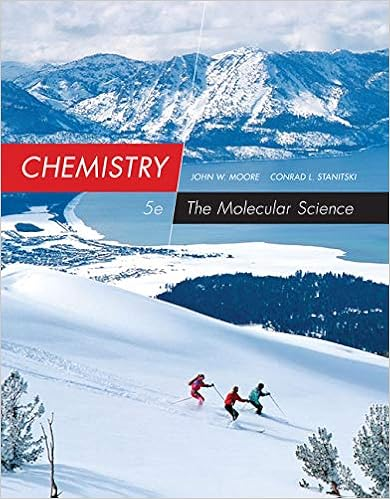A minus the mass of the beaker from experiment 2 g

• Lab Report
• rorpr
• 2
• 92% (65) 60 out of 65 people found this document helpful

This preview shows page 2 out of 2 pages.

We have textbook solutions for you!
The document you are viewing contains questions related to this textbook.The document you are viewing contains questions related to this textbook.
Chapter 2 / Exercise 129
Chemistry: The Molecular Science
Moore/StanitskiExpert Verified
(a minus the mass of the beaker from Experiment 2) (g) 2. Determine the amount of sodium chloride (NaCl) in the original mixture indirectly by adding the masses of ammonium chloride (experiment 1 e) and sand (experiment 2 c) together and subtracting the sum from the initial sample mass of 10 g. 2.62
3. Calculate the percent difference between the results from the two methods (directly and indirectly) from the following equation. You must show all work to receive full credit.
4. Based on your experimental results, which is the preferred method of separation?
5. What is the mass percent of each component in your mixture? To determine the mass percent, divide the mass of the component by the mass of the total 10.000g sample and multiply that result by 100. Make sure to show all work to receive full credit.
We have textbook solutions for you!
The document you are viewing contains questions related to this textbook.The document you are viewing contains questions related to this textbook.
Chapter 2 / Exercise 129
Chemistry: The Molecular Science
Moore/StanitskiExpert Verified
•••